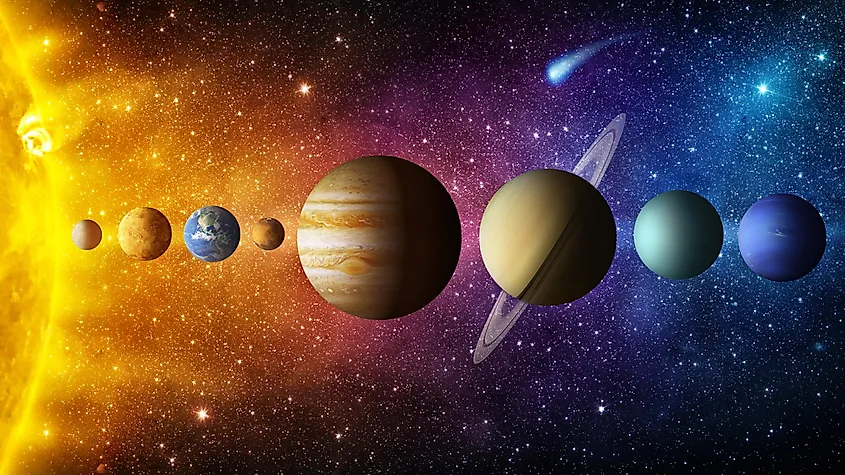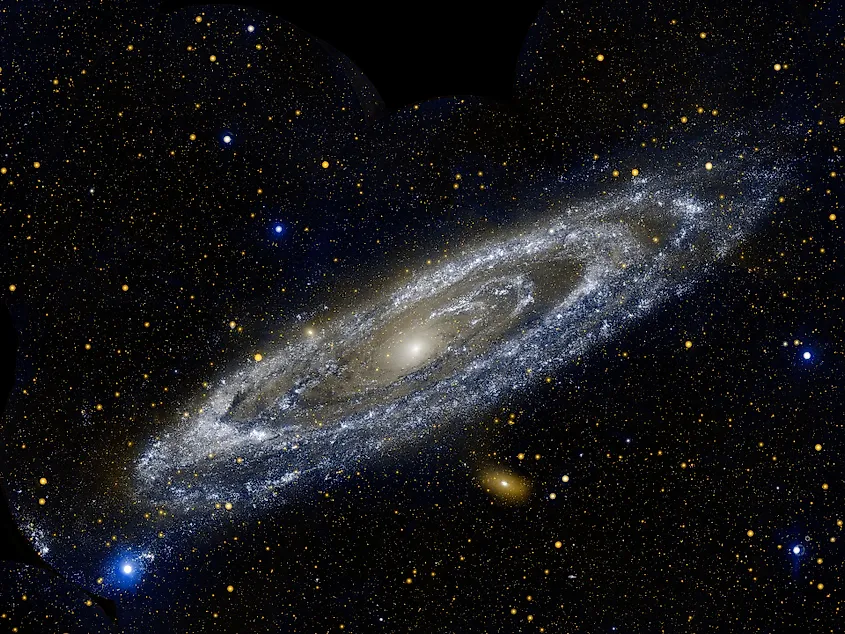# How Fast Is The Earth Moving?

From our everyday perspective, the Earth seems motionless. It was only a few hundred years ago that the idea of the Earth moving was seen as insane, yet now it is common knowledge. Despite the fact we do not feel the Earth move, our home world is currently spinning about its axis while also moving around the sun. Since Earth’s gravity has our feet firmly planted to the surface, we do not feel the Earth moving. Just how fast is the Earth moving?

## Rotational Speed

Everyone on Earth experiences the effects of Earth’s rotation. We have day and we have night, a cycle that repeats every 24-hours as the Earth spins about its axis. Since a full day is 24-hours long, that is the amount of time it takes for the Earth to make one full rotation. Interestingly, by knowing the amount of time it takes for Earth to rotate and the Earth’s circumference around the equator, you can easily calculate how fast Earth rotates. All you do is divide the circumference by the length of day. Earth’s circumference is about 24,898-miles (40,070-kilometres), which we can divide by 24-hours to get speed. Doing this, we get a rotational speed of 1,037-miles per hour (1,670-kilometres per hour). Since this number is calculated using the circumference at the equator, the Earth’s rotational speed is not uniform across the entire planet. As you move either north or south of the equator, the Earth’s rotational speed actually decreases.

## Orbital Speed

In addition to the fact that the Earth rotates about its axis, it is also orbiting the sun. Orbital speed is calculated through much the same way as rotational speed. You must know the amount of time it takes for Earth to orbit the sun and the total distance the Earth travels around the sun. Once you know these numbers, you simply divide distance by time to get speed. The amount of time it takes for the Earth to orbit the sun is one year, or about 365-days. Over the course of a year, the Earth travels 584-million miles (940-million kilometres). When we divide this number by 365-days, we get a speed of 1.6-million miles per day (2.6-million kilometres per day). Every hour that’s equal to 66,627-miles per hour (107,226-kilometres per hour).

## Solar System SpeedThe entire solar system is moving through the Milky Way Galaxy at a speed of 448,000-miles per hour

While we are all likely familiar with the fact that Earth spins and orbits the sun, these are only two forms of motion that the Earth experiences. In addition to those two values, the Earth is also being carried around the Milky Way with the sun. Like all the planets, the sun also has an orbit. In the case of the sun, it orbits the center of the Milky Way Galaxy, and it carries the entire solar system along with it. At this very moment, the solar system is moving through the Milky Way at a speed of 448,000-miles per hour (720,000-kilometres per hour). While that may seem extraordinarily fast, it will still take the sun about 230-million years to orbit the galaxy.

## Galactic SpeedThe Milky Way is moving towards the Andromeda Galaxy at a speed of 70-miles per second. Image credit: NASA

Every galaxy in the universe is moving through space, and that includes our home galaxy, the Milky Way. Our galaxy is currently speeding towards the Andromeda Galaxy at a rate of 70-miles per second (112-kilometres per second). Sometime in the next four to five-billion years, the Milky Way and Andromeda will start to collide, eventually merging together to become a single galaxy.

Share